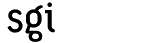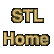# for_eachCategory: algorithms Component type: function

### Prototype

```template <class InputIterator, class UnaryFunction>
UnaryFunction for_each(InputIterator first, InputIterator last, UnaryFunction f);
```

### Description

For_each applies the function object f to each element in the range [first, last); f's return value, if any, is ignored. Applications are performed in forward order, i.e. from first to last. For_each returns the function object after it has been applied to each element. 

### Definition

Defined in the standard header algorithm, and in the nonstandard backward-compatibility header algo.h.

### Requirements on types

• InputIterator is a model of Input Iterator
• UnaryFunction is a model of Unary Function
• UnaryFunction does not apply any non-constant operation through its argument.
• InputIterator's value type is convertible to UnaryFunction's argument type.

### Preconditions

• [first, last) is a valid range.

### Complexity

Linear. Exactly last - first applications of UnaryFunction.

### Example

```template<class T> struct print : public unary_function<T, void>
{
print(ostream& out) : os(out), count(0) {}
void operator() (T x) { os << x << ' '; ++count; }
ostream& os;
int count;
};

int main()
{
int A[] = {1, 4, 2, 8, 5, 7};
const int N = sizeof(A) / sizeof(int);

print<int> P = for_each(A, A + N, print<int>(cout));
cout << endl << P.count << " objects printed." << endl;
}
```

### Notes

 This return value is sometimes useful, since a function object may have local state. It might, for example, count the number of times that it is called, or it might have a status flag to indicate whether or not a call succeeded.